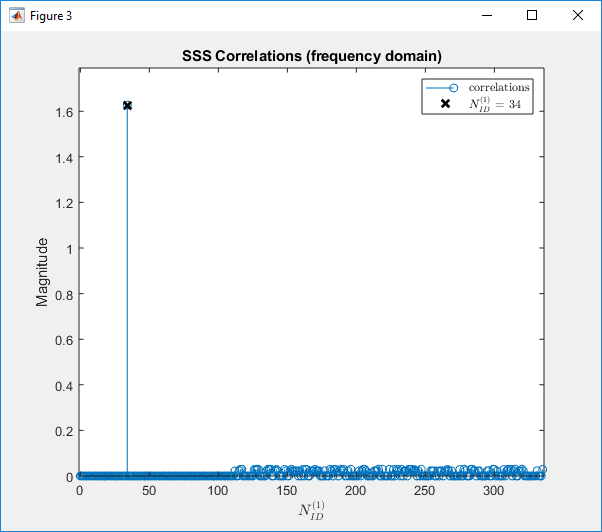Matlab Toolbox - 5G/NR

NR Synchronization in TDL Channel

NOTE :  It is required to have Matlab 5G Toolbox  to run this script. Matlab Version that I used for this page is 2019a v9.6, 5G Toolbox v1.1

I used the tutorial linked here almost as it is except a little bit of modifiation except a little bit of change in plotting part. So I would not put my own matlab code here.

Example 01 : TDL-C,Antenna 1x1, fc = 4e9, Velocity = 0.0, DelaySpread = 0

 % Burst configuration related to the burst structure itself: burst.BlockPattern = 'Case B'; burst.SSBPeriodicity = 20; burst.NFrame = 4; burst.SSBTransmitted = [1 1 1 1 1 1 1 1]; burst.NCellID = 102;   % number of Tx / Rx Antenna ntxants = 1; nrxants = 1;   % Configure channel velocity = 0.0; fc = 4e9; c = physconst('lightspeed'); fd = (velocity*1000/3600)/c*fc; channel = nrTDLChannel; channel.Seed = 24; channel.DelayProfile = 'TDL-C'; channel.DelaySpread =  0; channel.MaximumDopplerShift = fd; channel.MIMOCorrelation = 'Medium'; channel.Polarization = 'Cross-Polar'; channel.NumTransmitAntennas = ntxants; channel.NumReceiveAntennas = nrxants;   % Configure SNR for AWGN SNRdB = 50;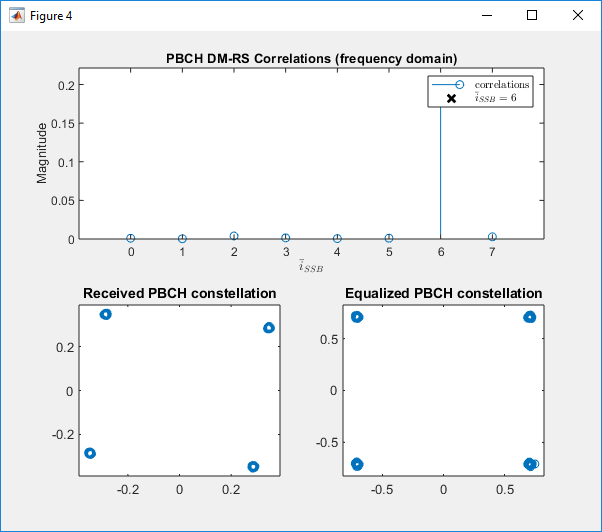Example 02 : TDL-C,Antenna 1x1, fc = 4e9, Velocity = 30.0, DelaySpread = 0

 % Burst configuration related to the burst structure itself: burst.BlockPattern = 'Case B'; burst.SSBPeriodicity = 20; burst.NFrame = 4; burst.SSBTransmitted = [1 1 1 1 1 1 1 1]; burst.NCellID = 102;   % number of Tx / Rx Antenna ntxants = 1; nrxants = 1;   % Configure channel velocity = 30.0; fc = 4e9; c = physconst('lightspeed'); fd = (velocity*1000/3600)/c*fc; channel = nrTDLChannel; channel.Seed = 24; channel.DelayProfile = 'TDL-C'; channel.DelaySpread =  0; channel.MaximumDopplerShift = fd; channel.MIMOCorrelation = 'Medium'; channel.Polarization = 'Cross-Polar'; channel.NumTransmitAntennas = ntxants; channel.NumReceiveAntennas = nrxants;   % Configure SNR for AWGN SNRdB = 50;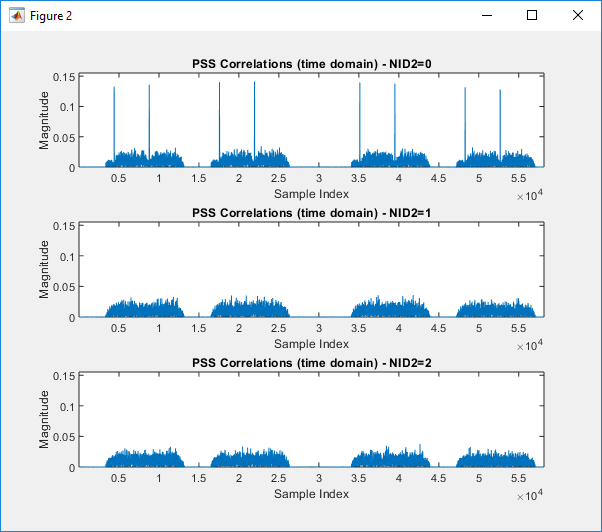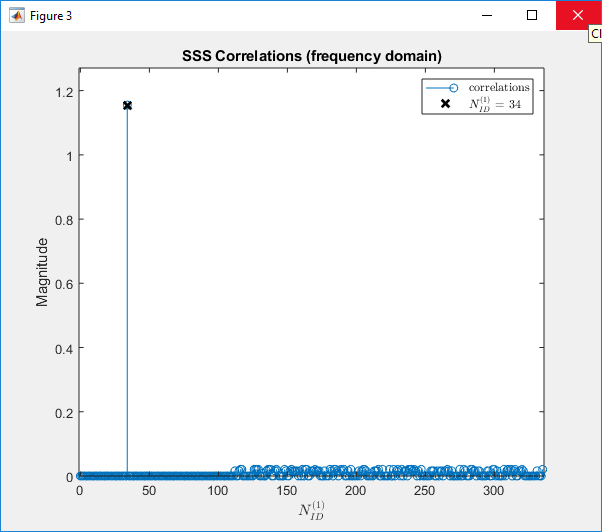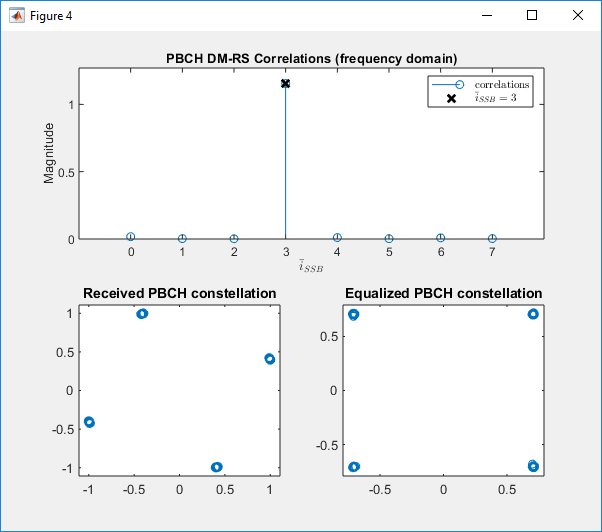Example 03 : TDL-C,Antenna 1x1, fc = 4e9, Velocity = 100.0, DelaySpread = 0

 % Burst configuration related to the burst structure itself: burst.BlockPattern = 'Case B'; burst.SSBPeriodicity = 20; burst.NFrame = 4; burst.SSBTransmitted = [1 1 1 1 1 1 1 1]; burst.NCellID = 102;   % number of Tx / Rx Antenna ntxants = 1; nrxants = 1;   % Configure channel velocity = 100.0; fc = 4e9; c = physconst('lightspeed'); fd = (velocity*1000/3600)/c*fc; channel = nrTDLChannel; channel.Seed = 24; channel.DelayProfile = 'TDL-C'; channel.DelaySpread =  0; channel.MaximumDopplerShift = fd; channel.MIMOCorrelation = 'Medium'; channel.Polarization = 'Cross-Polar'; channel.NumTransmitAntennas = ntxants; channel.NumReceiveAntennas = nrxants;   % Configure SNR for AWGN SNRdB = 50;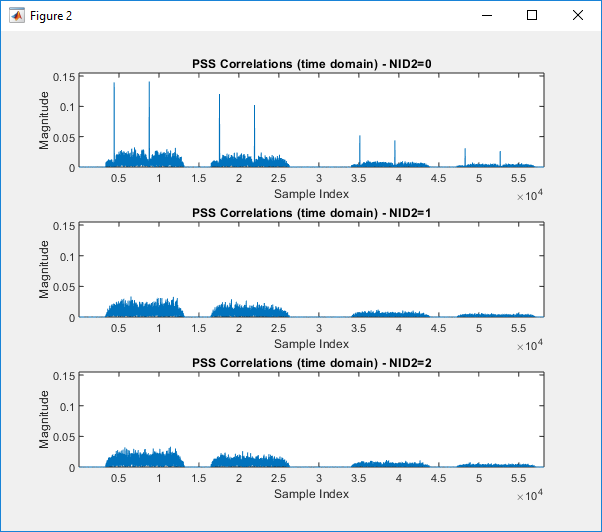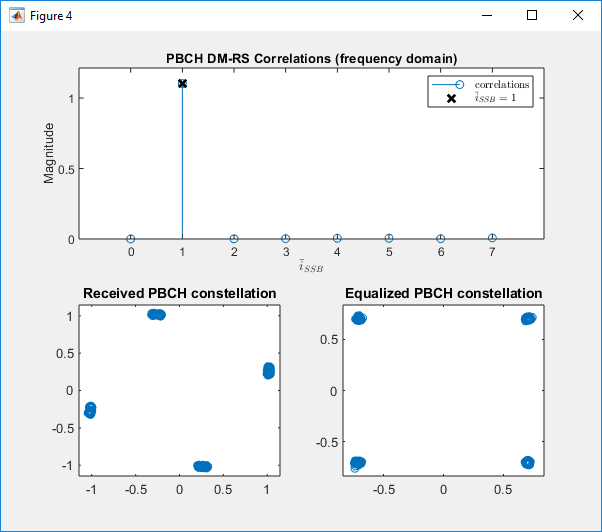Example 04 : TDL-C,Antenna 1x1, fc = 4e9, Velocity = 200.0, DelaySpread = 0

 % Burst configuration related to the burst structure itself: burst.BlockPattern = 'Case B'; burst.SSBPeriodicity = 20; burst.NFrame = 4; burst.SSBTransmitted = [1 1 1 1 1 1 1 1]; burst.NCellID = 102;   % number of Tx / Rx Antenna ntxants = 1; nrxants = 1;   % Configure channel velocity = 200.0; fc = 4e9; c = physconst('lightspeed'); fd = (velocity*1000/3600)/c*fc; channel = nrTDLChannel; channel.Seed = 24; channel.DelayProfile = 'TDL-C'; channel.DelaySpread =  0; channel.MaximumDopplerShift = fd; channel.MIMOCorrelation = 'Medium'; channel.Polarization = 'Cross-Polar'; channel.NumTransmitAntennas = ntxants; channel.NumReceiveAntennas = nrxants;   % Configure SNR for AWGN SNRdB = 50;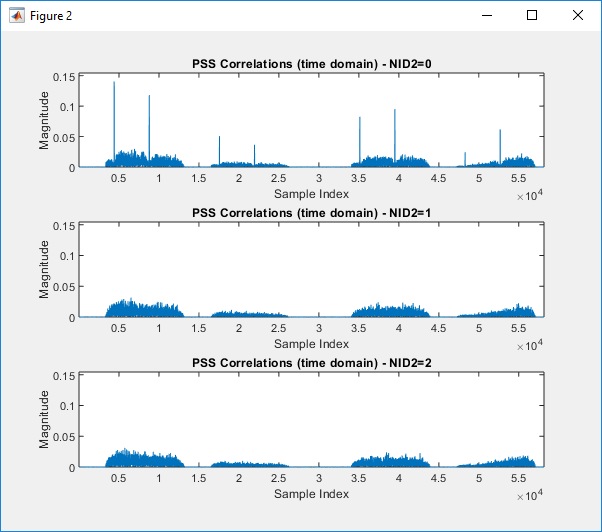Example 05 : TDL-C,Antenna 1x1, fc = 4e9, Velocity = 300.0, DelaySpread = 0

 % Burst configuration related to the burst structure itself: burst.BlockPattern = 'Case B'; burst.SSBPeriodicity = 20; burst.NFrame = 4; burst.SSBTransmitted = [1 1 1 1 1 1 1 1]; burst.NCellID = 102;   % number of Tx / Rx Antenna ntxants = 1; nrxants = 1;   % Configure channel velocity = 300.0; fc = 4e9; c = physconst('lightspeed'); fd = (velocity*1000/3600)/c*fc; channel = nrTDLChannel; channel.Seed = 24; channel.DelayProfile = 'TDL-C'; channel.DelaySpread =  0; channel.MaximumDopplerShift = fd; channel.MIMOCorrelation = 'Medium'; channel.Polarization = 'Cross-Polar'; channel.NumTransmitAntennas = ntxants; channel.NumReceiveAntennas = nrxants;   % Configure SNR for AWGN SNRdB = 50;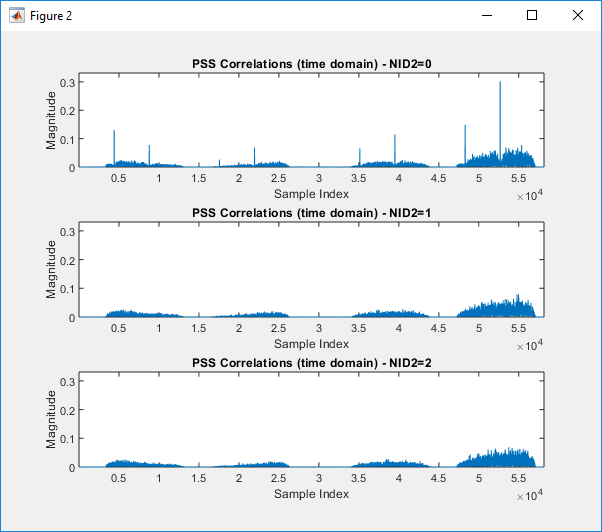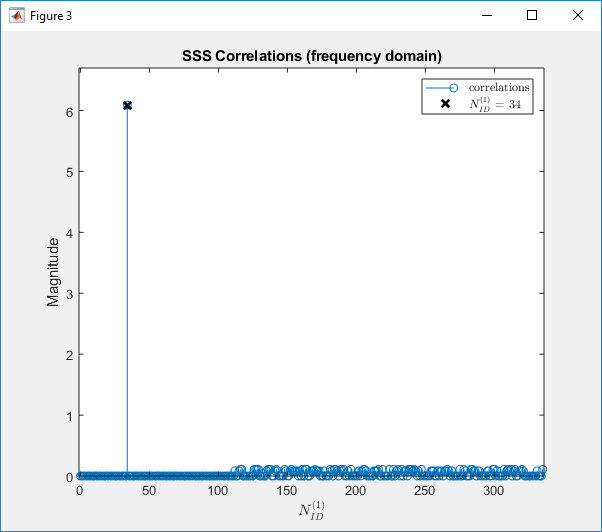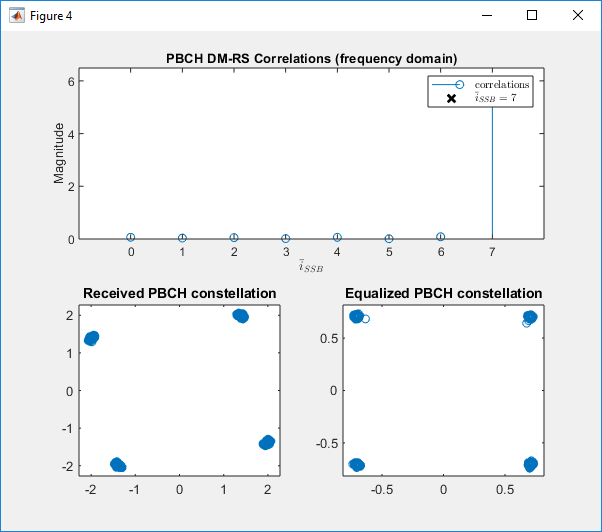Example 06 : TDL-C,Antenna 1x1, fc = 4e9, Velocity = 0.0, DelaySpread = 300e-9

 % Burst configuration related to the burst structure itself: burst.BlockPattern = 'Case B'; burst.SSBPeriodicity = 20; burst.NFrame = 4; burst.SSBTransmitted = [1 1 1 1 1 1 1 1]; burst.NCellID = 102;   % number of Tx / Rx Antenna ntxants = 1; nrxants = 1;   % Configure channel velocity = 0.0; fc = 4e9; c = physconst('lightspeed'); fd = (velocity*1000/3600)/c*fc; channel = nrTDLChannel; channel.Seed = 24; channel.DelayProfile = 'TDL-C'; channel.DelaySpread =  300e-9; channel.MaximumDopplerShift = fd; channel.MIMOCorrelation = 'Medium'; channel.Polarization = 'Cross-Polar'; channel.NumTransmitAntennas = ntxants; channel.NumReceiveAntennas = nrxants;   % Configure SNR for AWGN SNRdB = 50;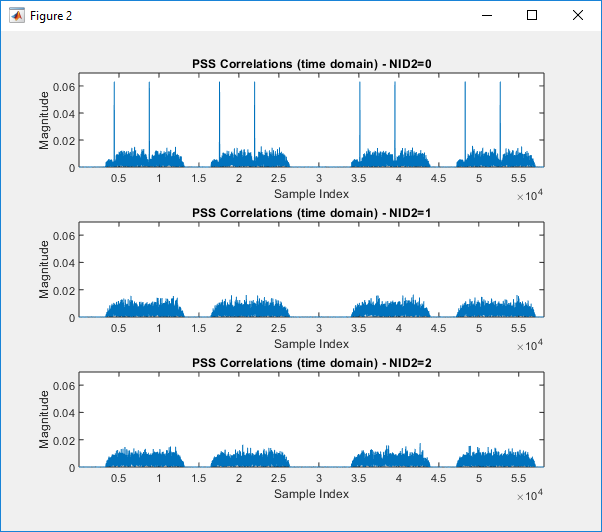Example 07 : TDL-C,Antenna 1x1, fc = 4e9, Velocity = 0.0, DelaySpread = 100e-9

 % Burst configuration related to the burst structure itself: burst.BlockPattern = 'Case B'; burst.SSBPeriodicity = 20; burst.NFrame = 4; burst.SSBTransmitted = [1 1 1 1 1 1 1 1]; burst.NCellID = 102;   % number of Tx / Rx Antenna ntxants = 1; nrxants = 1;   % Configure channel velocity = 0.0; fc = 4e9; c = physconst('lightspeed'); fd = (velocity*1000/3600)/c*fc; channel = nrTDLChannel; channel.Seed = 24; channel.DelayProfile = 'TDL-C'; channel.DelaySpread =  100e-9; channel.MaximumDopplerShift = fd; channel.MIMOCorrelation = 'Medium'; channel.Polarization = 'Cross-Polar'; channel.NumTransmitAntennas = ntxants; channel.NumReceiveAntennas = nrxants;   % Configure SNR for AWGN SNRdB = 50;Example 08 : TDL-C,Antenna 1x1, fc = 4e9, Velocity = 0.0, DelaySpread = 200e-9

 % Burst configuration related to the burst structure itself: burst.BlockPattern = 'Case B'; burst.SSBPeriodicity = 20; burst.NFrame = 4; burst.SSBTransmitted = [1 1 1 1 1 1 1 1]; burst.NCellID = 102;   % number of Tx / Rx Antenna ntxants = 1; nrxants = 1;   % Configure channel velocity = 0.0; fc = 4e9; c = physconst('lightspeed'); fd = (velocity*1000/3600)/c*fc; channel = nrTDLChannel; channel.Seed = 24; channel.DelayProfile = 'TDL-C'; channel.DelaySpread =  200e-9; channel.MaximumDopplerShift = fd; channel.MIMOCorrelation = 'Medium'; channel.Polarization = 'Cross-Polar'; channel.NumTransmitAntennas = ntxants; channel.NumReceiveAntennas = nrxants;   % Configure SNR for AWGN SNRdB = 50;Example 09 : TDL-C,Antenna 1x1, fc = 4e9, Velocity = 0.0, DelaySpread = 300e-9

 % Burst configuration related to the burst structure itself: burst.BlockPattern = 'Case B'; burst.SSBPeriodicity = 20; burst.NFrame = 4; burst.SSBTransmitted = [1 1 1 1 1 1 1 1]; burst.NCellID = 102;   % number of Tx / Rx Antenna ntxants = 1; nrxants = 1;   % Configure channel velocity = 0.0; fc = 4e9; c = physconst('lightspeed'); fd = (velocity*1000/3600)/c*fc; channel = nrTDLChannel; channel.Seed = 24; channel.DelayProfile = 'TDL-C'; channel.DelaySpread =  300e-9; channel.MaximumDopplerShift = fd; channel.MIMOCorrelation = 'Medium'; channel.Polarization = 'Cross-Polar'; channel.NumTransmitAntennas = ntxants; channel.NumReceiveAntennas = nrxants;   % Configure SNR for AWGN SNRdB = 50;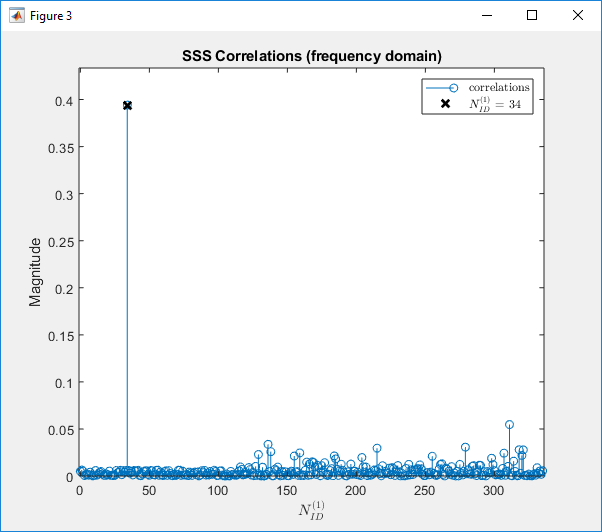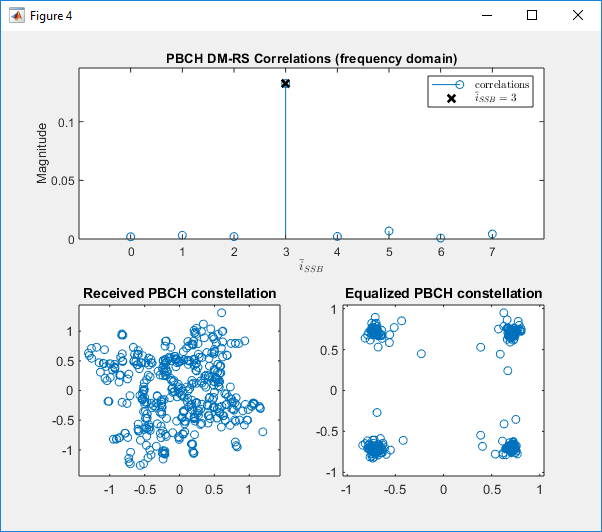Example 10 : TDL-C,Antenna 1x1, fc = 4e9, Velocity = 0.0, DelaySpread = 400e-9

 % Burst configuration related to the burst structure itself: burst.BlockPattern = 'Case B'; burst.SSBPeriodicity = 20; burst.NFrame = 4; burst.SSBTransmitted = [1 1 1 1 1 1 1 1]; burst.NCellID = 102;   % number of Tx / Rx Antenna ntxants = 1; nrxants = 1;   % Configure channel velocity = 0.0; fc = 4e9; c = physconst('lightspeed'); fd = (velocity*1000/3600)/c*fc; channel = nrTDLChannel; channel.Seed = 24; channel.DelayProfile = 'TDL-C'; channel.DelaySpread =  400e-9; channel.MaximumDopplerShift = fd; channel.MIMOCorrelation = 'Medium'; channel.Polarization = 'Cross-Polar'; channel.NumTransmitAntennas = ntxants; channel.NumReceiveAntennas = nrxants;   % Configure SNR for AWGN SNRdB = 50;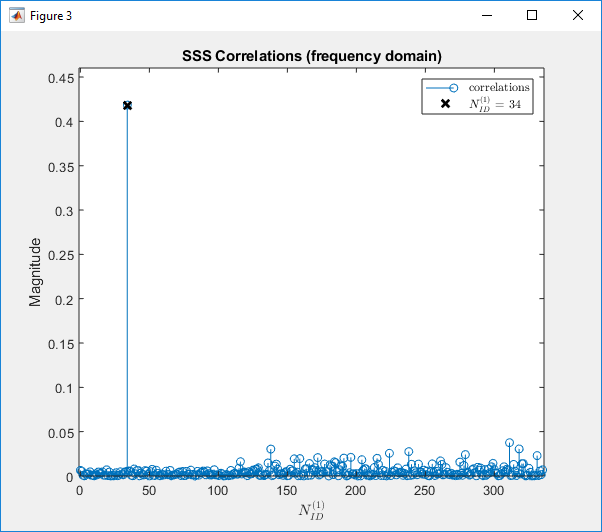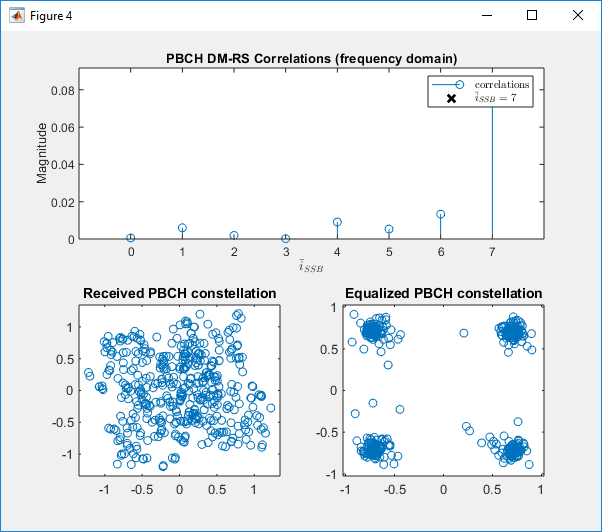Example 11 : TDL-C,Antenna 1x1, fc = 4e9, Velocity = 30.0, DelaySpread = 300e-9

 % Burst configuration related to the burst structure itself: burst.BlockPattern = 'Case B'; burst.SSBPeriodicity = 20; burst.NFrame = 4; burst.SSBTransmitted = [1 1 1 1 1 1 1 1]; burst.NCellID = 102;   % number of Tx / Rx Antenna ntxants = 1; nrxants = 1;   % Configure channel velocity = 30.0; fc = 4e9; c = physconst('lightspeed'); fd = (velocity*1000/3600)/c*fc; channel = nrTDLChannel; channel.Seed = 24; channel.DelayProfile = 'TDL-C'; channel.DelaySpread =  300e-9; channel.MaximumDopplerShift = fd; channel.MIMOCorrelation = 'Medium'; channel.Polarization = 'Cross-Polar'; channel.NumTransmitAntennas = ntxants; channel.NumReceiveAntennas = nrxants;   % Configure SNR for AWGN SNRdB = 50;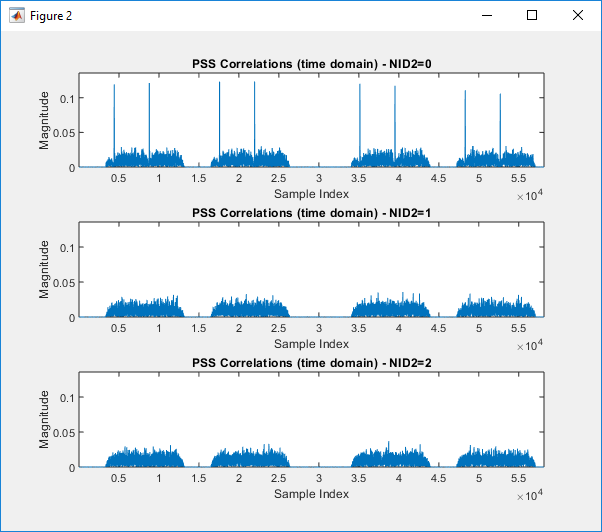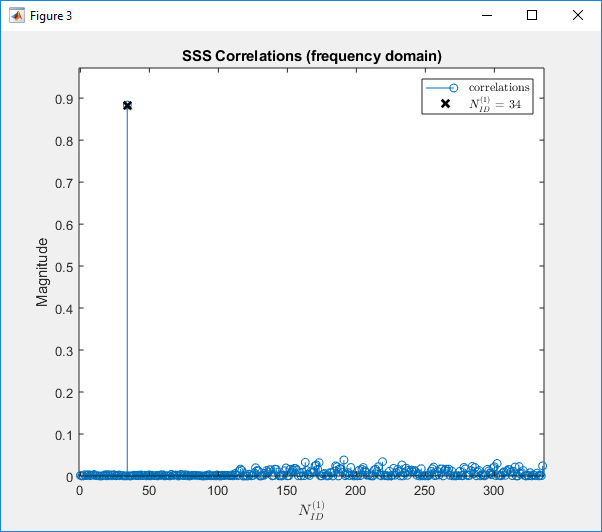Example 12 : TDL-C,Antenna 2x2 fc = 4e9, Velocity = 0.0, DelaySpread = 0

 % Burst configuration related to the burst structure itself: burst.BlockPattern = 'Case B'; burst.SSBPeriodicity = 20; burst.NFrame = 4; burst.SSBTransmitted = [1 1 1 1 1 1 1 1]; burst.NCellID = 102;     % number of Tx / Rx Antenna ntxants = 2; nrxants = 2;   % Configure channel velocity = 0.0; fc = 4e9; c = physconst('lightspeed'); fd = (velocity*1000/3600)/c*fc; channel = nrTDLChannel; channel.Seed = 24; channel.DelayProfile = 'TDL-C'; channel.DelaySpread =  0; channel.MaximumDopplerShift = fd; channel.MIMOCorrelation = 'Medium'; channel.Polarization = 'Cross-Polar'; channel.NumTransmitAntennas = ntxants; channel.NumReceiveAntennas = nrxants;   % Configure SNR for AWGN SNRdB = 50;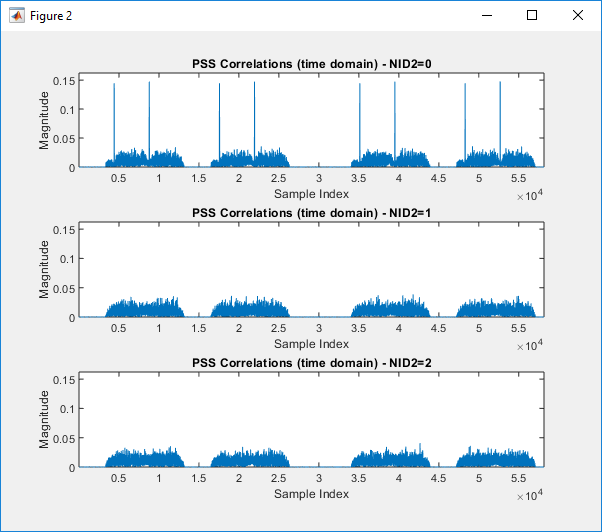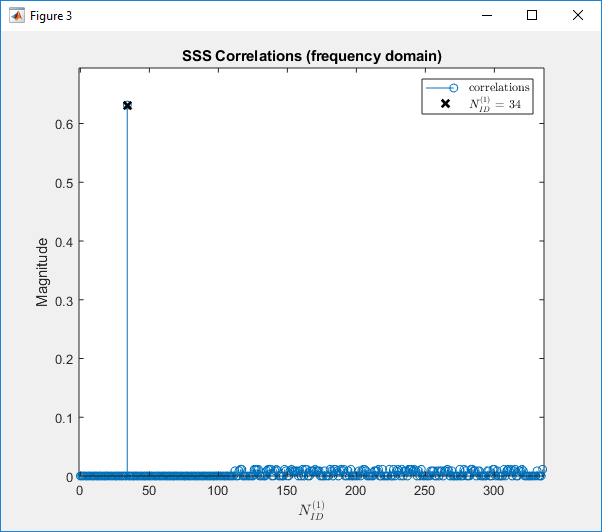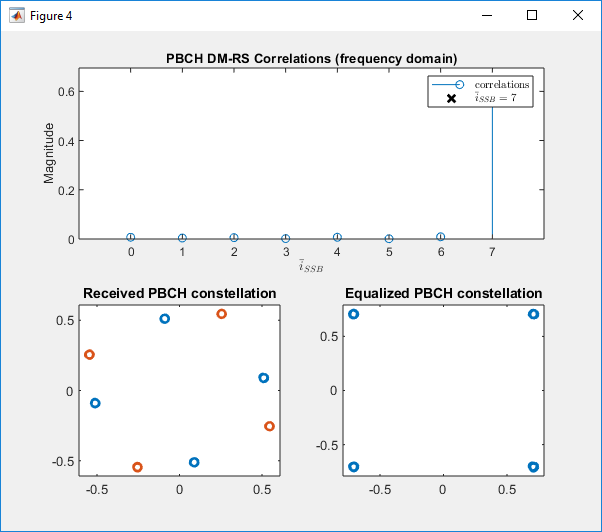Example 13 : TDL-C,Antenna 2x2, fc = 4e9, Velocity = 30.0, DelaySpread = 0

 % Burst configuration related to the burst structure itself: burst.BlockPattern = 'Case B'; burst.SSBPeriodicity = 20; burst.NFrame = 4; burst.SSBTransmitted = [1 1 1 1 1 1 1 1]; burst.NCellID = 102;   % number of Tx / Rx Antenna ntxants = 2; nrxants = 2;   % Configure channel velocity = 30.0; fc = 4e9; c = physconst('lightspeed'); fd = (velocity*1000/3600)/c*fc; channel = nrTDLChannel; channel.Seed = 24; channel.DelayProfile = 'TDL-C'; channel.DelaySpread =  0; channel.MaximumDopplerShift = fd; channel.MIMOCorrelation = 'Medium'; channel.Polarization = 'Cross-Polar'; channel.NumTransmitAntennas = ntxants; channel.NumReceiveAntennas = nrxants;   % Configure SNR for AWGN SNRdB = 50;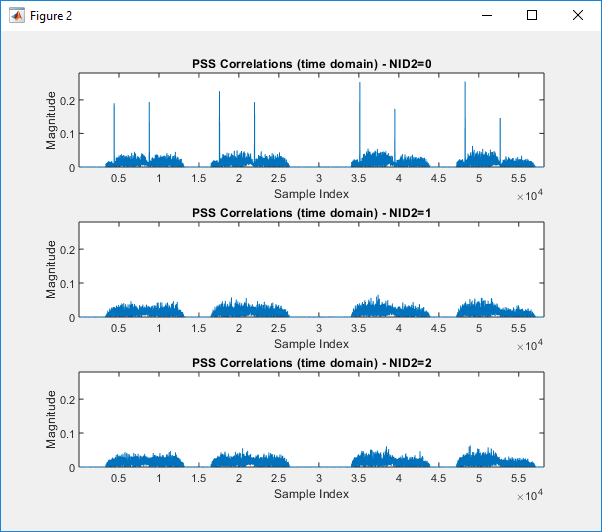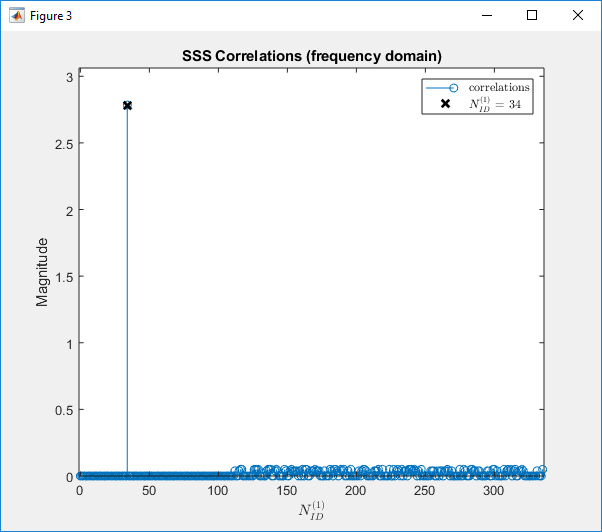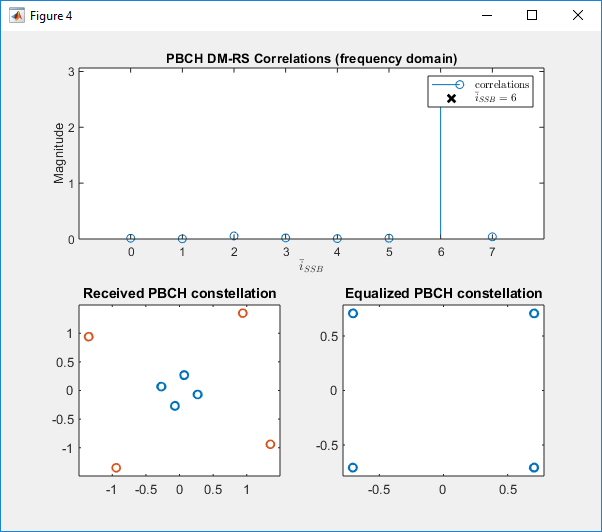Example 14 : TDL-C,Antenna 2x2, fc = 4e9, Velocity = 30.0, DelaySpread = 300e-9

 % Burst configuration related to the burst structure itself: burst.BlockPattern = 'Case B'; burst.SSBPeriodicity = 20; burst.NFrame = 4; burst.SSBTransmitted = [1 1 1 1 1 1 1 1]; burst.NCellID = 102;   % number of Tx / Rx Antenna ntxants = 2; nrxants = 2;   % Configure channel velocity = 30.0; fc = 4e9; c = physconst('lightspeed'); fd = (velocity*1000/3600)/c*fc; channel = nrTDLChannel; channel.Seed = 24; channel.DelayProfile = 'TDL-C'; channel.DelaySpread =  300e-9; channel.MaximumDopplerShift = fd; channel.MIMOCorrelation = 'Medium'; channel.Polarization = 'Cross-Polar'; channel.NumTransmitAntennas = ntxants; channel.NumReceiveAntennas = nrxants;   % Configure SNR for AWGN SNRdB = 50;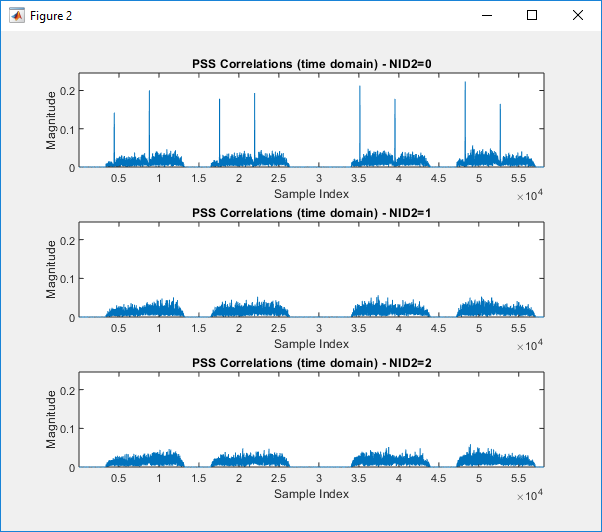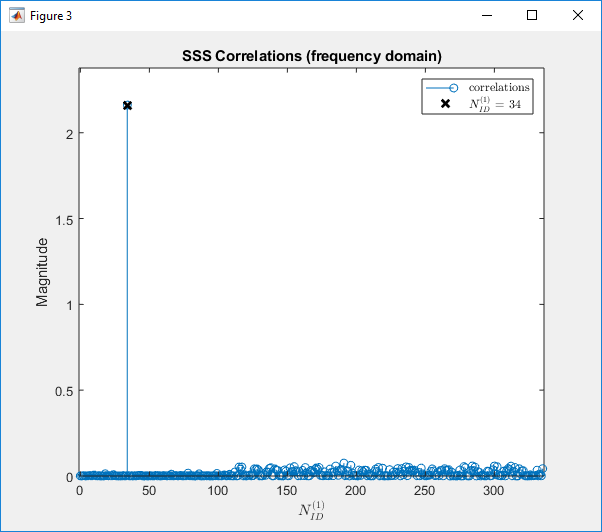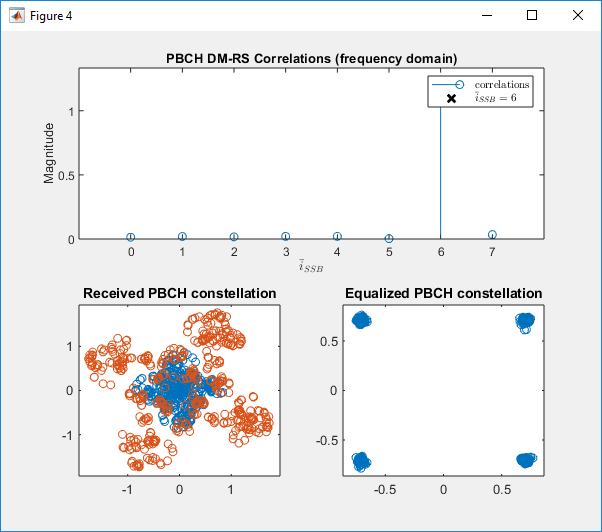Example 15 : TDL-C,Antenna 4x2, fc = 4e9, Velocity = 0.0, DelaySpread = 0

 % Burst configuration related to the burst structure itself: burst.BlockPattern = 'Case B'; burst.SSBPeriodicity = 20; burst.NFrame = 4; burst.SSBTransmitted = [1 1 1 1 1 1 1 1]; burst.NCellID = 102;     % number of Tx / Rx Antenna ntxants = 4; nrxants = 2;   % Configure channel velocity = 0.0; fc = 4e9; c = physconst('lightspeed'); fd = (velocity*1000/3600)/c*fc; channel = nrTDLChannel; channel.Seed = 24; channel.DelayProfile = 'TDL-C'; channel.DelaySpread =  0; channel.MaximumDopplerShift = fd; channel.MIMOCorrelation = 'Medium'; channel.Polarization = 'Cross-Polar'; channel.NumTransmitAntennas = ntxants; channel.NumReceiveAntennas = nrxants;   % Configure SNR for AWGN SNRdB = 50;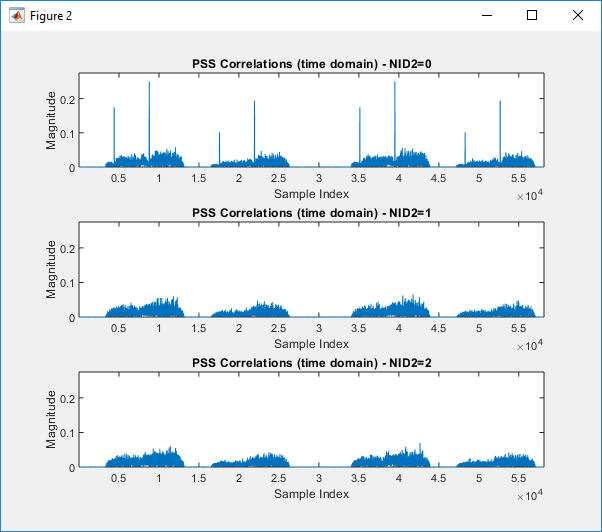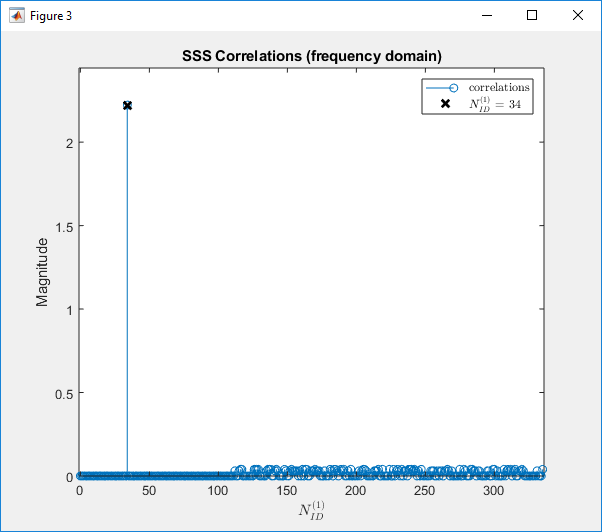Example 16 : TDL-C,Antenna 4x2, fc = 4e9, Velocity = 30.0, DelaySpread = 0

 % Burst configuration related to the burst structure itself: burst.BlockPattern = 'Case B'; burst.SSBPeriodicity = 20; burst.NFrame = 4; burst.SSBTransmitted = [1 1 1 1 1 1 1 1]; burst.NCellID = 102;     % number of Tx / Rx Antenna ntxants = 4; nrxants = 2;   % Configure channel velocity = 30.0; fc = 4e9; c = physconst('lightspeed'); fd = (velocity*1000/3600)/c*fc; channel = nrTDLChannel; channel.Seed = 24; channel.DelayProfile = 'TDL-C'; channel.DelaySpread =  0; channel.MaximumDopplerShift = fd; channel.MIMOCorrelation = 'Medium'; channel.Polarization = 'Cross-Polar'; channel.NumTransmitAntennas = ntxants; channel.NumReceiveAntennas = nrxants;   % Configure SNR for AWGN SNRdB = 50;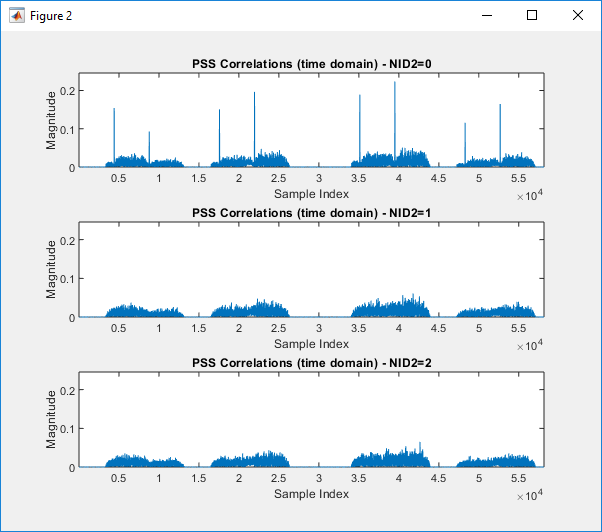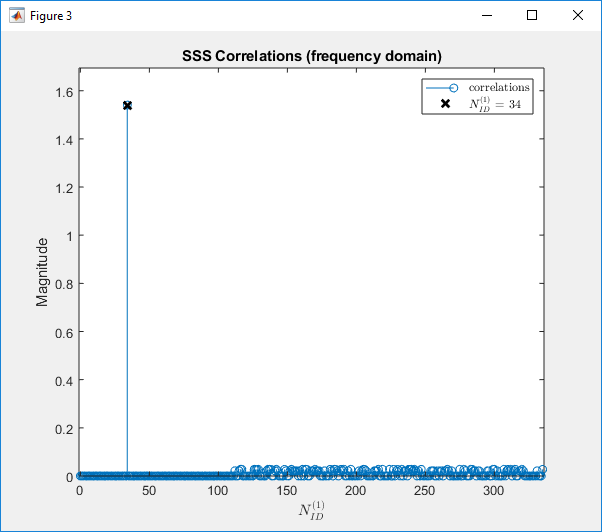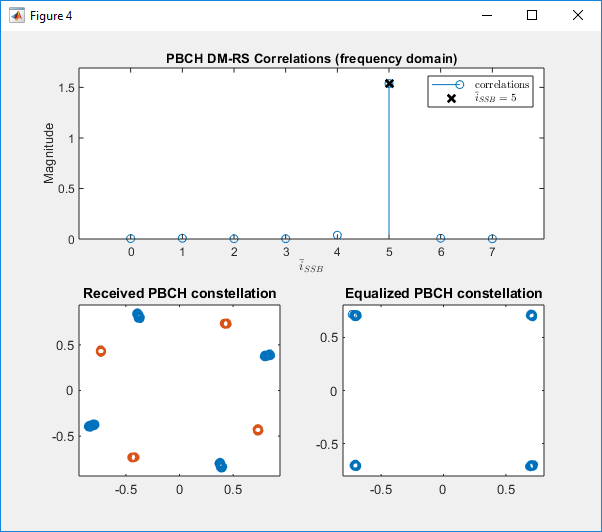Example 17 : TDL-C,Antenna 4x2, fc = 4e9, Velocity = 30.0, DelaySpread = 300e-9

 % Burst configuration related to the burst structure itself: burst.BlockPattern = 'Case B'; burst.SSBPeriodicity = 20; burst.NFrame = 4; burst.SSBTransmitted = [1 1 1 1 1 1 1 1]; burst.NCellID = 102;     % number of Tx / Rx Antenna ntxants = 4; nrxants = 2;   % Configure channel velocity = 30.0; fc = 4e9; c = physconst('lightspeed'); fd = (velocity*1000/3600)/c*fc; channel = nrTDLChannel; channel.Seed = 24; channel.DelayProfile = 'TDL-C'; channel.DelaySpread =  300e-9; channel.MaximumDopplerShift = fd; channel.MIMOCorrelation = 'Medium'; channel.Polarization = 'Cross-Polar'; channel.NumTransmitAntennas = ntxants; channel.NumReceiveAntennas = nrxants;   % Configure SNR for AWGN SNRdB = 50;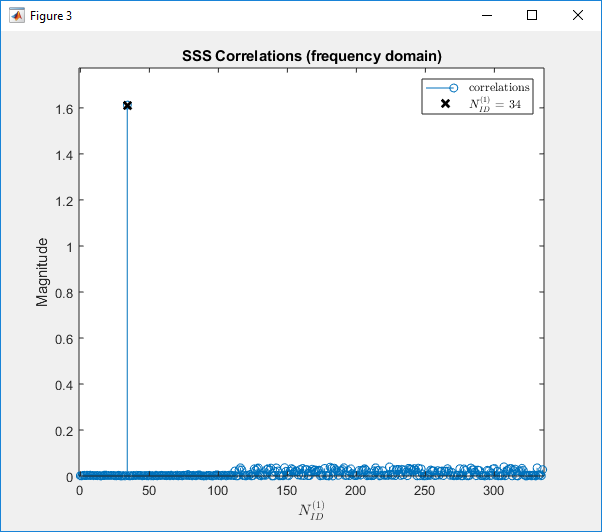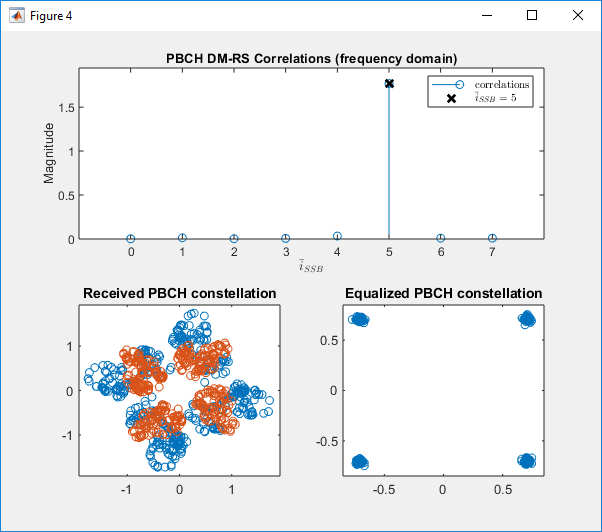Example 18 : TDL-C,Antenna 4x4, fc = 4e9, Velocity = 0.0, DelaySpread = 0

 % Burst configuration related to the burst structure itself: burst.BlockPattern = 'Case B'; burst.SSBPeriodicity = 20; burst.NFrame = 4; burst.SSBTransmitted = [1 1 1 1 1 1 1 1]; burst.NCellID = 102;     % number of Tx / Rx Antenna ntxants = 4; nrxants = 4;   % Configure channel velocity = 0.0; fc = 4e9; c = physconst('lightspeed'); fd = (velocity*1000/3600)/c*fc; channel = nrTDLChannel; channel.Seed = 24; channel.DelayProfile = 'TDL-C'; channel.DelaySpread =  0; channel.MaximumDopplerShift = fd; channel.MIMOCorrelation = 'Medium'; channel.Polarization = 'Cross-Polar'; channel.NumTransmitAntennas = ntxants; channel.NumReceiveAntennas = nrxants;   % Configure SNR for AWGN SNRdB = 50;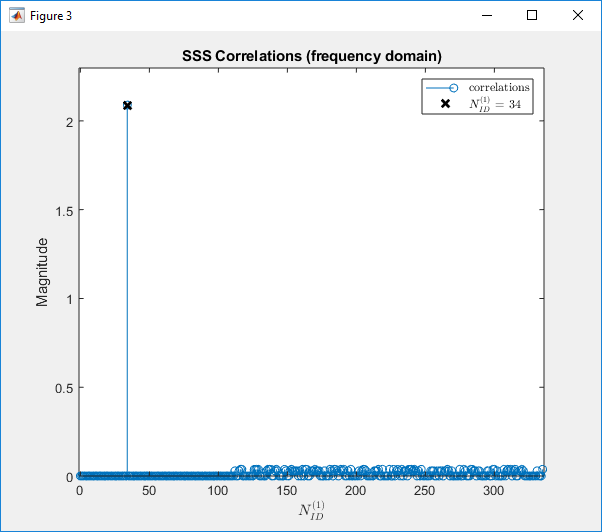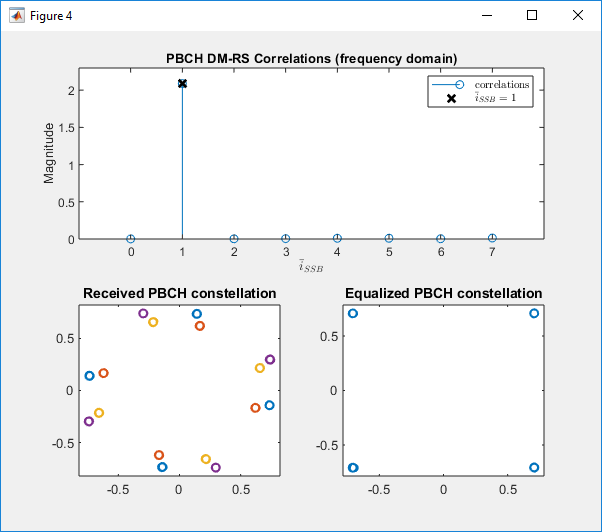Example 19 : TDL-C,Antenna 4x4, fc = 4e9, Velocity = 30.0, DelaySpread = 0

 % Burst configuration related to the burst structure itself: burst.BlockPattern = 'Case B'; burst.SSBPeriodicity = 20; burst.NFrame = 4; burst.SSBTransmitted = [1 1 1 1 1 1 1 1]; burst.NCellID = 102;     % number of Tx / Rx Antenna ntxants = 4; nrxants = 4;   % Configure channel velocity = 30.0; fc = 4e9; c = physconst('lightspeed'); fd = (velocity*1000/3600)/c*fc; channel = nrTDLChannel; channel.Seed = 24; channel.DelayProfile = 'TDL-C'; channel.DelaySpread =  0; channel.MaximumDopplerShift = fd; channel.MIMOCorrelation = 'Medium'; channel.Polarization = 'Cross-Polar'; channel.NumTransmitAntennas = ntxants; channel.NumReceiveAntennas = nrxants;   % Configure SNR for AWGN SNRdB = 50;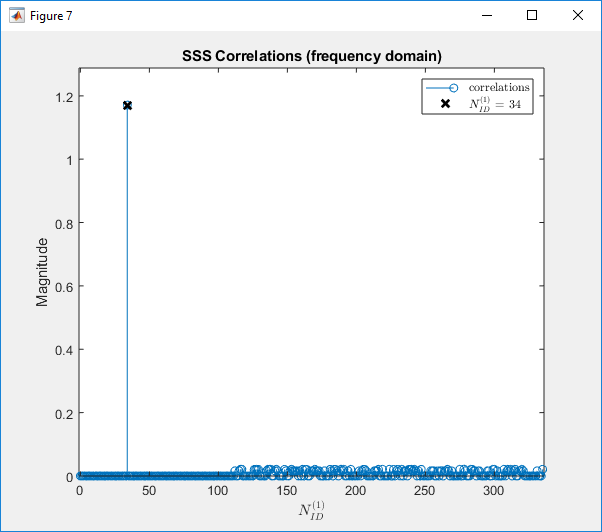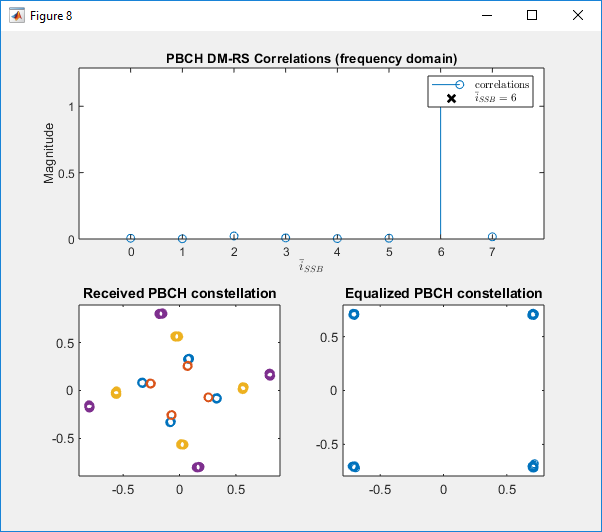Example 20 : TDL-C,Antenna 4x4, fc = 4e9, Velocity = 30.0, DelaySpread = 300e-9

 % Burst configuration related to the burst structure itself: burst.BlockPattern = 'Case B'; burst.SSBPeriodicity = 20; burst.NFrame = 4; burst.SSBTransmitted = [1 1 1 1 1 1 1 1]; burst.NCellID = 102;     % number of Tx / Rx Antenna ntxants = 4; nrxants = 4;   % Configure channel velocity = 30.0; fc = 4e9; c = physconst('lightspeed'); fd = (velocity*1000/3600)/c*fc; channel = nrTDLChannel; channel.Seed = 24; channel.DelayProfile = 'TDL-C'; channel.DelaySpread =  300e-9; channel.MaximumDopplerShift = fd; channel.MIMOCorrelation = 'Medium'; channel.Polarization = 'Cross-Polar'; channel.NumTransmitAntennas = ntxants; channel.NumReceiveAntennas = nrxants;   % Configure SNR for AWGN SNRdB = 50;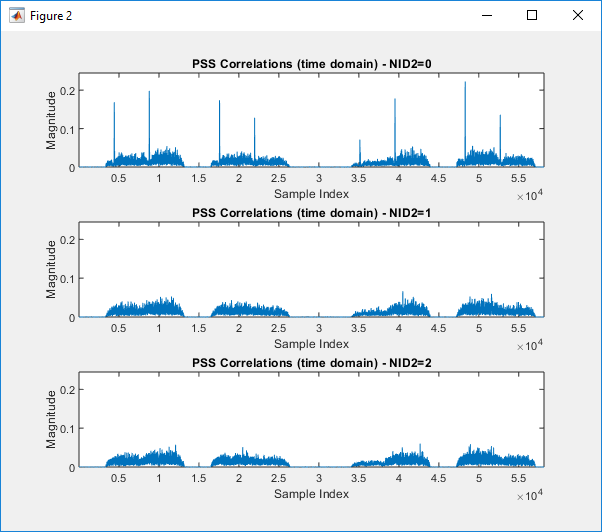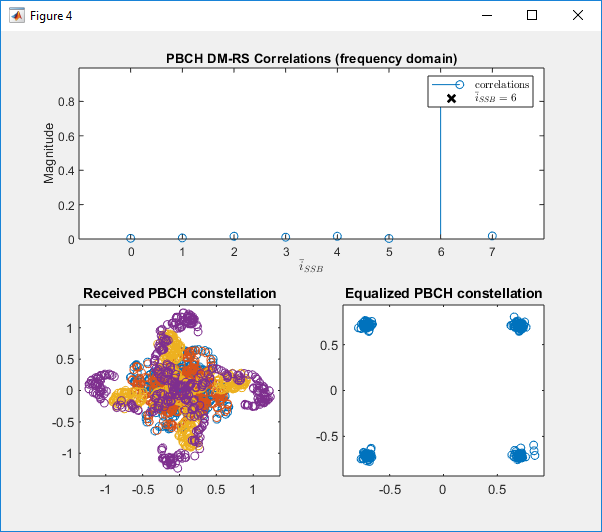Example 21 : TDL-C,Antenna 2x2, fc = 4e9, Velocity = 0.0, DelaySpread = 0

 % Burst configuration related to the burst structure itself: burst.BlockPattern = 'Case B'; burst.SSBPeriodicity = 20; burst.NFrame = 4; burst.SSBTransmitted = [1 1 1 1 1 1 1 1]; burst.NCellID = 102;     % number of Tx / Rx Antenna ntxants = 2; nrxants = 2;   % Configure channel velocity = 0.0; fc = 4e9; c = physconst('lightspeed'); fd = (velocity*1000/3600)/c*fc; channel = nrTDLChannel; channel.Seed = 24; channel.DelayProfile = 'TDL-C'; channel.DelaySpread =  0; channel.MaximumDopplerShift = fd; channel.MIMOCorrelation = 'Medium'; channel.Polarization = 'Cross-Polar'; channel.NumTransmitAntennas = ntxants; channel.NumReceiveAntennas = nrxants;   % Configure SNR for AWGN SNRdB = 20;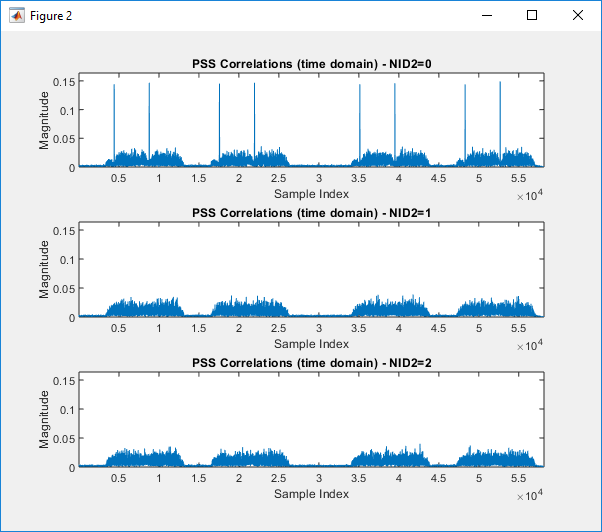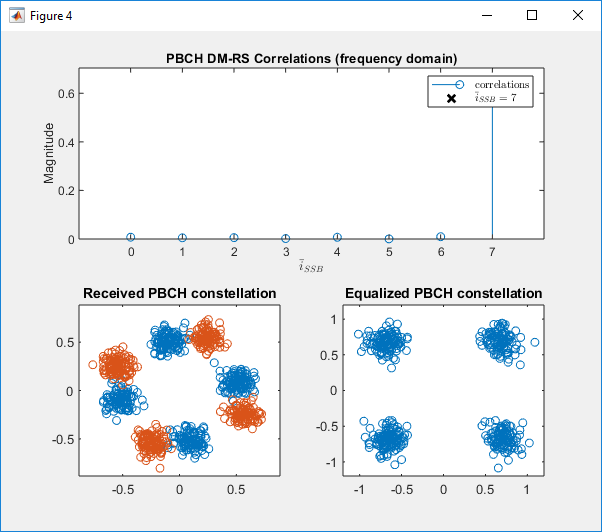Example 22 : TDL-C,Antenna 2x2, fc = 4e9, Velocity = 0.0, DelaySpread = 0

 % Burst configuration related to the burst structure itself: burst.BlockPattern = 'Case B'; burst.SSBPeriodicity = 20; burst.NFrame = 4; burst.SSBTransmitted = [1 1 1 1 1 1 1 1]; burst.NCellID = 102;     % number of Tx / Rx Antenna ntxants = 2; nrxants = 2;   % Configure channel velocity = 0.0; fc = 4e9; c = physconst('lightspeed'); fd = (velocity*1000/3600)/c*fc; channel = nrTDLChannel; channel.Seed = 24; channel.DelayProfile = 'TDL-C'; channel.DelaySpread =  0; channel.MaximumDopplerShift = fd; channel.MIMOCorrelation = 'Medium'; channel.Polarization = 'Cross-Polar'; channel.NumTransmitAntennas = ntxants; channel.NumReceiveAntennas = nrxants;   % Configure SNR for AWGN SNRdB = 20;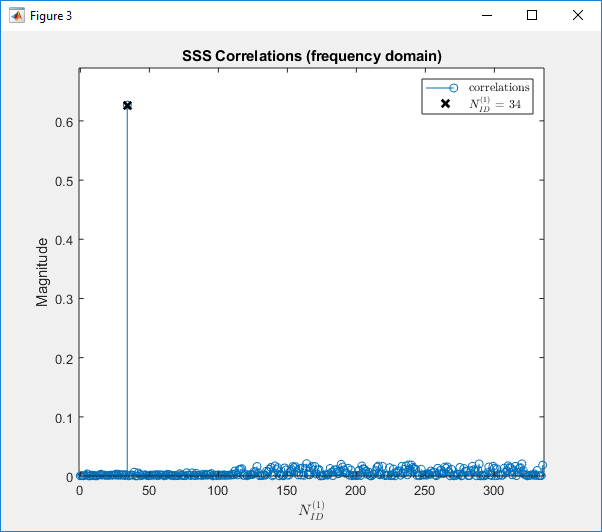Example 23 : TDL-A,Antenna 1x1, fc = 4e9, Velocity = 0.0, DelaySpread = 50e-9

 % Burst configuration related to the burst structure itself: burst.BlockPattern = 'Case B'; burst.SSBPeriodicity = 20; burst.NFrame = 4; burst.SSBTransmitted = [1 1 1 1 1 1 1 1]; burst.NCellID = 102;   % number of Tx / Rx Antenna ntxants = 1; nrxants = 1;   % Configure channel velocity = 0.0; fc = 4e9; c = physconst('lightspeed'); fd = (velocity*1000/3600)/c*fc; channel = nrTDLChannel; channel.Seed = 24; channel.DelayProfile = 'TDL-A'; channel.DelaySpread =  50e-9; channel.MaximumDopplerShift = fd; channel.MIMOCorrelation = 'Medium'; channel.Polarization = 'Cross-Polar'; channel.NumTransmitAntennas = ntxants; channel.NumReceiveAntennas = nrxants;   % Configure SNR for AWGN SNRdB = 50;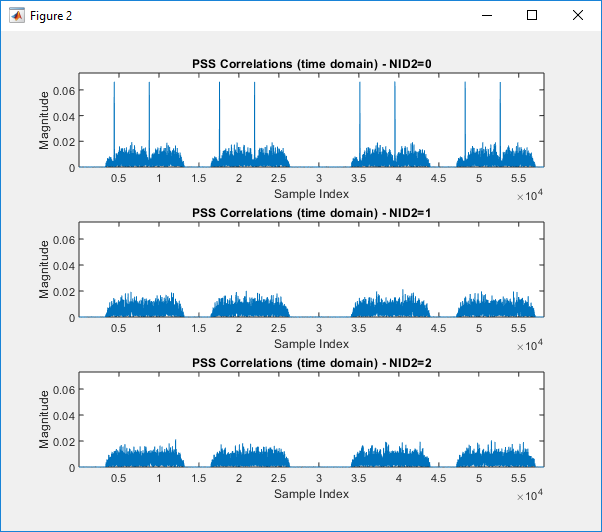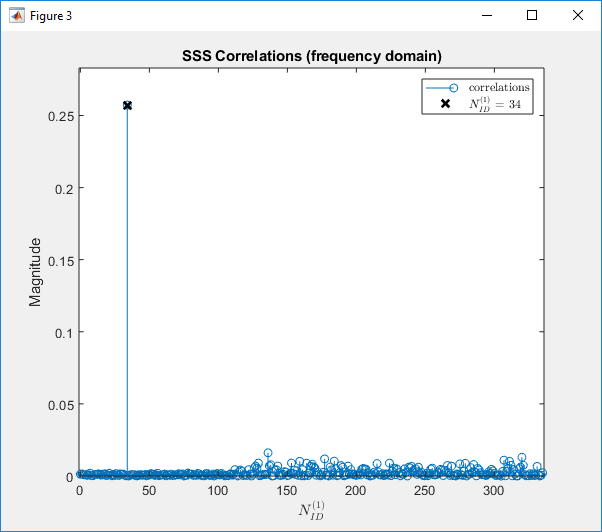Example 24 : TDL-B,Antenna 1x1, fc = 4e9, Velocity = 0.0, DelaySpread = 50e-9

 % Burst configuration related to the burst structure itself: burst.BlockPattern = 'Case B'; burst.SSBPeriodicity = 20; burst.NFrame = 4; burst.SSBTransmitted = [1 1 1 1 1 1 1 1]; burst.NCellID = 102;   % number of Tx / Rx Antenna ntxants = 1; nrxants = 1;   % Configure channel velocity = 0.0; fc = 4e9; c = physconst('lightspeed'); fd = (velocity*1000/3600)/c*fc; channel = nrTDLChannel; channel.Seed = 24; channel.DelayProfile = 'TDL-B; channel.DelaySpread =  50e-9; channel.MaximumDopplerShift = fd; channel.MIMOCorrelation = 'Medium'; channel.Polarization = 'Cross-Polar'; channel.NumTransmitAntennas = ntxants; channel.NumReceiveAntennas = nrxants;   % Configure SNR for AWGN SNRdB = 50;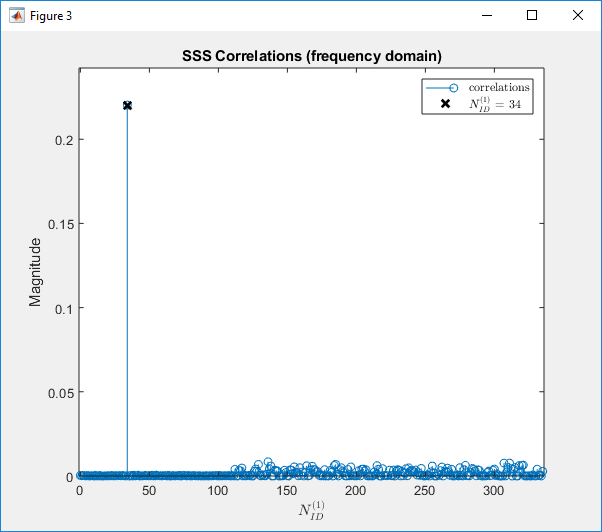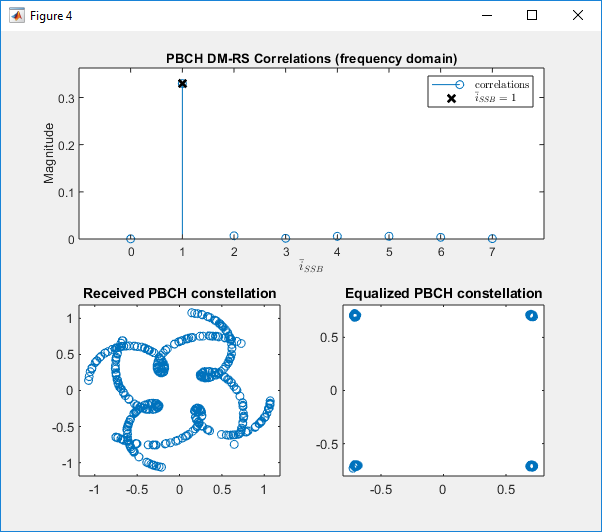Example 25 : TDL-C,Antenna 1x1, fc = 4e9, Velocity = 0.0, DelaySpread = 50e-9

 % Burst configuration related to the burst structure itself: burst.BlockPattern = 'Case B'; burst.SSBPeriodicity = 20; burst.NFrame = 4; burst.SSBTransmitted = [1 1 1 1 1 1 1 1]; burst.NCellID = 102;   % number of Tx / Rx Antenna ntxants = 1; nrxants = 1;   % Configure channel velocity = 0.0; fc = 4e9; c = physconst('lightspeed'); fd = (velocity*1000/3600)/c*fc; channel = nrTDLChannel; channel.Seed = 24; channel.DelayProfile = 'TDL-C'; channel.DelaySpread =  50e-9; channel.MaximumDopplerShift = fd; channel.MIMOCorrelation = 'Medium'; channel.Polarization = 'Cross-Polar'; channel.NumTransmitAntennas = ntxants; channel.NumReceiveAntennas = nrxants;   % Configure SNR for AWGN SNRdB = 50;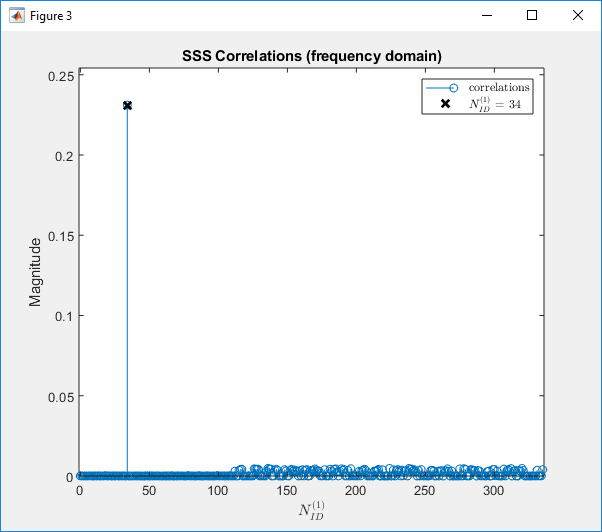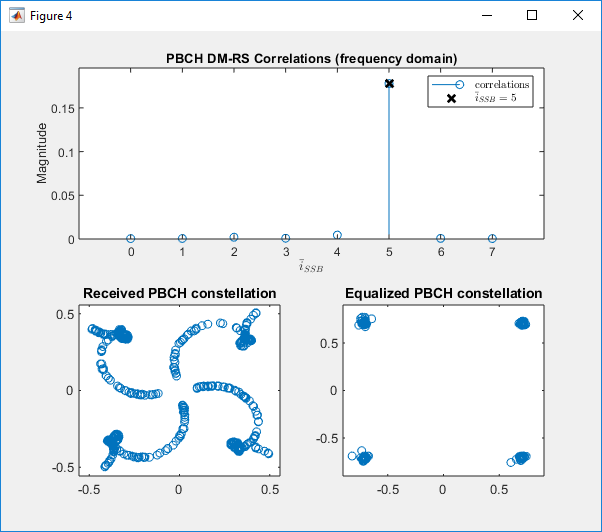Example 26 : TDL-D,Antenna 1x1, fc = 4e9, Velocity = 0.0, DelaySpread = 50e-9

 % Burst configuration related to the burst structure itself: burst.BlockPattern = 'Case B'; burst.SSBPeriodicity = 20; burst.NFrame = 4; burst.SSBTransmitted = [1 1 1 1 1 1 1 1]; burst.NCellID = 102;   % number of Tx / Rx Antenna ntxants = 1; nrxants = 1;   % Configure channel velocity = 0.0; fc = 4e9; c = physconst('lightspeed'); fd = (velocity*1000/3600)/c*fc; channel = nrTDLChannel; channel.Seed = 24; channel.DelayProfile = 'TDL-D'; channel.DelaySpread =  50e-9; channel.MaximumDopplerShift = fd; channel.MIMOCorrelation = 'Medium'; channel.Polarization = 'Cross-Polar'; channel.NumTransmitAntennas = ntxants; channel.NumReceiveAntennas = nrxants;   % Configure SNR for AWGN SNRdB = 50;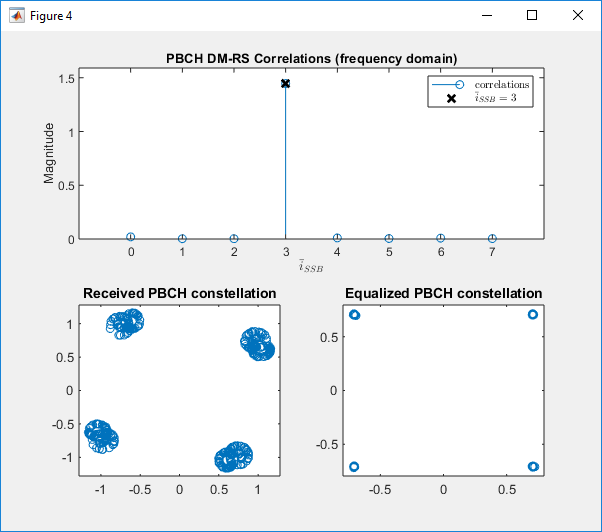Example 27 : TDL-E,Antenna 1x1, fc = 4e9, Velocity = 0.0, DelaySpread = 50e-9

 % Burst configuration related to the burst structure itself: burst.BlockPattern = 'Case B'; burst.SSBPeriodicity = 20; burst.NFrame = 4; burst.SSBTransmitted = [1 1 1 1 1 1 1 1]; burst.NCellID = 102;   % number of Tx / Rx Antenna ntxants = 1; nrxants = 1;   % Configure channel velocity = 0.0; fc = 4e9; c = physconst('lightspeed'); fd = (velocity*1000/3600)/c*fc; channel = nrTDLChannel; channel.Seed = 24; channel.DelayProfile = 'TDL-E'; channel.DelaySpread =  50e-9; channel.MaximumDopplerShift = fd; channel.MIMOCorrelation = 'Medium'; channel.Polarization = 'Cross-Polar'; channel.NumTransmitAntennas = ntxants; channel.NumReceiveAntennas = nrxants;   % Configure SNR for AWGN SNRdB = 50;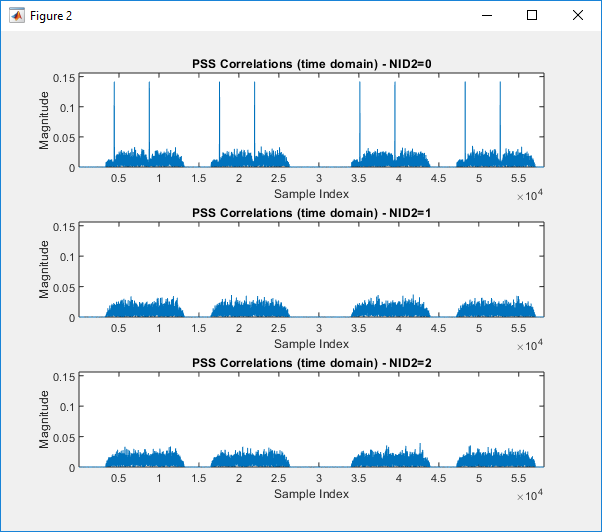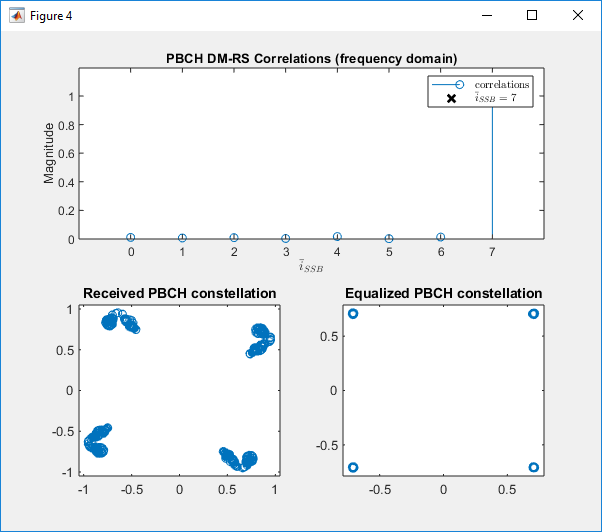Example 28 : TDL-C,Antenna 2x2, fc = 4e9, Velocity = 0.0, DelaySpread = 50e-9, MIMOCorrelation = Low

 % Burst configuration related to the burst structure itself: burst.BlockPattern = 'Case B'; burst.SSBPeriodicity = 20; burst.NFrame = 4; burst.SSBTransmitted = [1 1 1 1 1 1 1 1]; burst.NCellID = 102;     % number of Tx / Rx Antenna ntxants = 2; nrxants = 2;   % Configure channel velocity = 0.0; fc = 4e9; c = physconst('lightspeed'); fd = (velocity*1000/3600)/c*fc; channel = nrTDLChannel; channel.Seed = 24; channel.DelayProfile = 'TDL-C'; channel.DelaySpread =  50e-9; channel.MaximumDopplerShift = fd; channel.MIMOCorrelation = 'Low'; channel.Polarization = 'Cross-Polar'; channel.NumTransmitAntennas = ntxants; channel.NumReceiveAntennas = nrxants;   % Configure SNR for AWGN SNRdB = 50;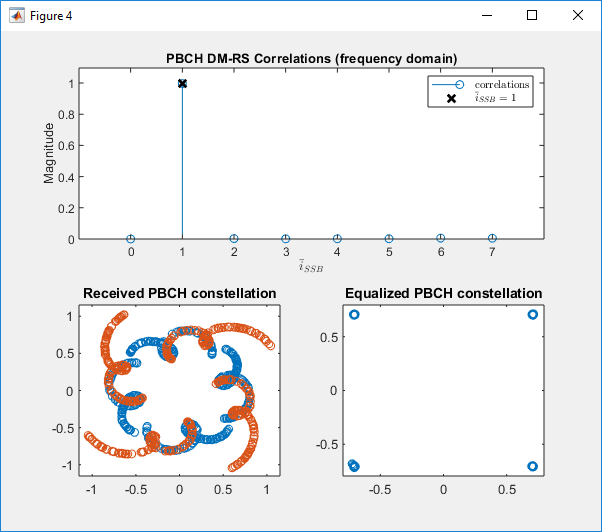Example 29 : TDL-C,Antenna 2x2, fc = 4e9, Velocity = 0.0, DelaySpread = 50e-9, MIMOCorrelation = Medium

 % Burst configuration related to the burst structure itself: burst.BlockPattern = 'Case B'; burst.SSBPeriodicity = 20; burst.NFrame = 4; burst.SSBTransmitted = [1 1 1 1 1 1 1 1]; burst.NCellID = 102;     % number of Tx / Rx Antenna ntxants = 2; nrxants = 2;   % Configure channel velocity = 0.0; fc = 4e9; c = physconst('lightspeed'); fd = (velocity*1000/3600)/c*fc; channel = nrTDLChannel; channel.Seed = 24; channel.DelayProfile = 'TDL-C'; channel.DelaySpread =  50e-9; channel.MaximumDopplerShift = fd; channel.MIMOCorrelation = 'Medium'; channel.Polarization = 'Cross-Polar'; channel.NumTransmitAntennas = ntxants; channel.NumReceiveAntennas = nrxants;   % Configure SNR for AWGN SNRdB = 50;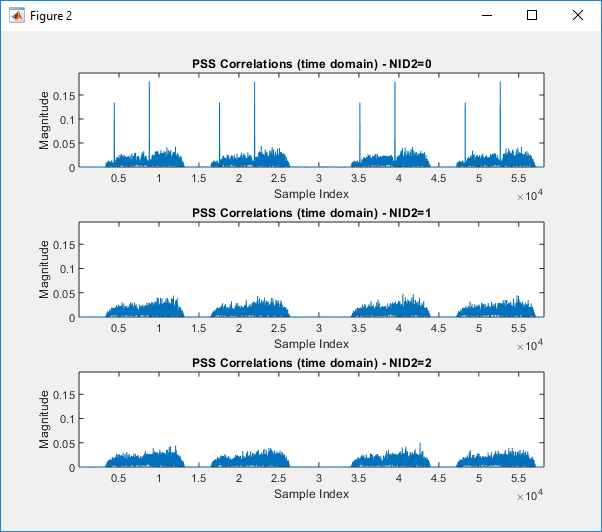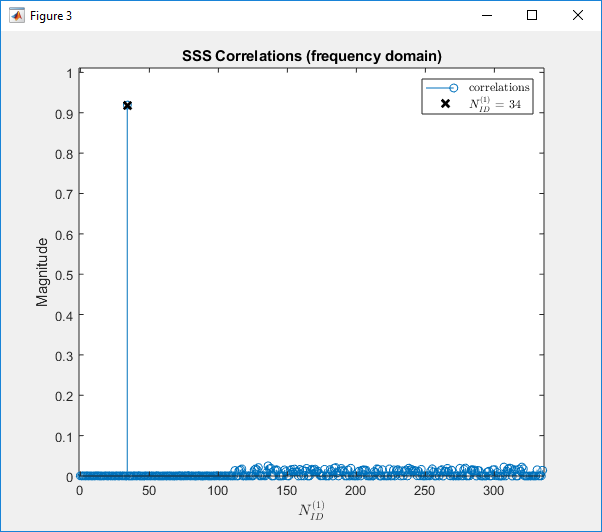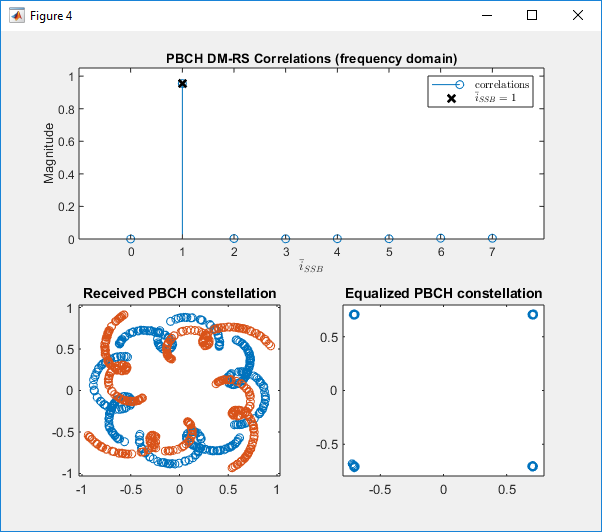Example 30 : TDL-C,Antenna 2x2, fc = 4e9, Velocity = 0.0, DelaySpread = 50e-9, MIMOCorrelation = Medium-A

 % Burst configuration related to the burst structure itself: burst.BlockPattern = 'Case B'; burst.SSBPeriodicity = 20; burst.NFrame = 4; burst.SSBTransmitted = [1 1 1 1 1 1 1 1]; burst.NCellID = 102;     % number of Tx / Rx Antenna ntxants = 2; nrxants = 2;   % Configure channel velocity = 0.0; fc = 4e9; c = physconst('lightspeed'); fd = (velocity*1000/3600)/c*fc; channel = nrTDLChannel; channel.Seed = 24; channel.DelayProfile = 'TDL-C'; channel.DelaySpread =  50e-9; channel.MaximumDopplerShift = fd; channel.MIMOCorrelation = Medium-A'; channel.Polarization = 'Cross-Polar'; channel.NumTransmitAntennas = ntxants; channel.NumReceiveAntennas = nrxants;   % Configure SNR for AWGN SNRdB = 50;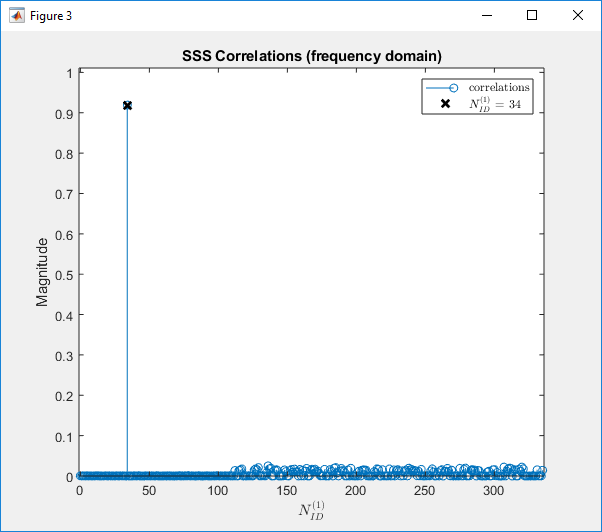Example 31 : TDL-C,Antenna 2x2, fc = 4e9, Velocity = 0.0, DelaySpread = 50e-9,Polarization='Co-Polar'

 % Burst configuration related to the burst structure itself: burst.BlockPattern = 'Case B'; burst.SSBPeriodicity = 20; burst.NFrame = 4; burst.SSBTransmitted = [1 1 1 1 1 1 1 1]; burst.NCellID = 102;     % number of Tx / Rx Antenna ntxants = 2; nrxants = 2;   % Configure channel velocity = 0.0; fc = 4e9; c = physconst('lightspeed'); fd = (velocity*1000/3600)/c*fc; channel = nrTDLChannel; channel.Seed = 24; channel.DelayProfile = 'TDL-C'; channel.DelaySpread =  50e-9; channel.MaximumDopplerShift = fd; channel.MIMOCorrelation = 'Medium'; channel.Polarization = 'Co-Polar'; channel.NumTransmitAntennas = ntxants; channel.NumReceiveAntennas = nrxants;   % Configure SNR for AWGN SNRdB = 50;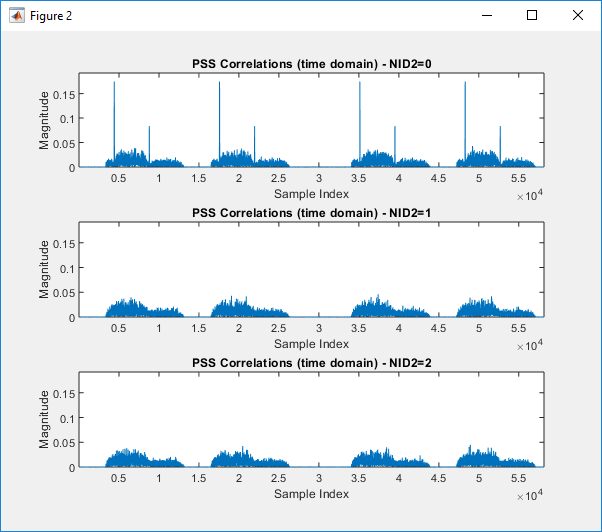Example 32 : Custom,Antenna 2x2, fc = 4e9, Velocity = 0.0, DelaySpread = 50e-9

 % Burst configuration related to the burst structure itself: burst.BlockPattern = 'Case B'; burst.SSBPeriodicity = 20; burst.NFrame = 4; burst.SSBTransmitted = [1 1 1 1 1 1 1 1]; burst.NCellID = 102;     % number of Tx / Rx Antenna ntxants = 2; nrxants = 2;   velocity = 0.0; fc = 4e9; c = physconst('lightspeed') fd = (velocity*1000/3600)/c*fc;   tapDelay = [0.0000 0.3819 0.4025 0.5868 0.4610 ...             0.5375 0.6708 0.5750 0.7618 1.5375 ...             1.8978 2.2242 2.1718 2.4942 2.5119 ...             3.0582 4.0810 4.4579 4.5695 4.7966 ...             5.0066 5.3043 9.6586]; tapGain = [-13.4 0 -2.2 -4 -6 ...            -8.2 -9.9 -10.5 -7.5 -15.9 ...            -6.6 -16.7 -12.4 -15.2 -10.8 ...            -11.3 -12.7 -16.2 -18.3 -18.9 ...            -16.6 -19.9 -29.7];           channel = nrTDLChannel; channel.Seed = 24; channel.DelayProfile = 'Custom'; channel.AveragePathGains = tapGain; channel.PathDelays = tapDelay * 1e-9; %channel.DelaySpread =  50e-9; channel.MaximumDopplerShift = fd; channel.MIMOCorrelation = 'Medium'; channel.Polarization = 'Cross-Polar'; channel.NumTransmitAntennas = ntxants; channel.NumReceiveAntennas = nrxants; tdl = info(channel);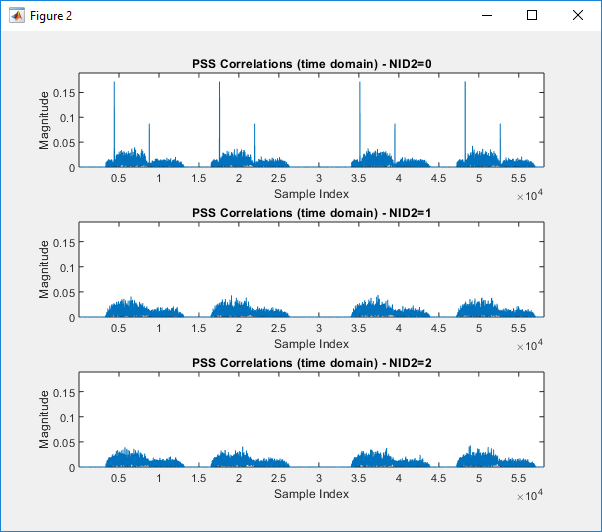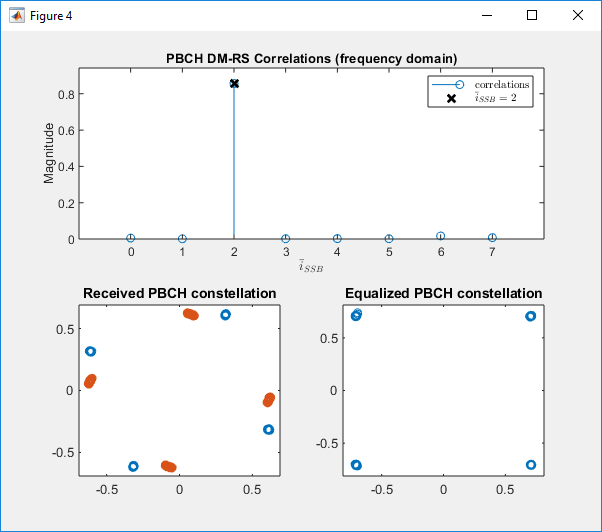Example 33 : TDL-C,Antenna 1x1, fc = 4e9, Velocity = 300.0, DelaySpread = 50e-9

 % Burst configuration related to the burst structure itself: burst.BlockPattern = 'Case B'; burst.SSBPeriodicity = 20; burst.NFrame = 4; burst.SSBTransmitted = [1 1 1 1 1 1 1 1]; burst.NCellID = 102;     velocity = 300.0; fc = 4e9; c = physconst('lightspeed') fd = (velocity*1000/3600)/c*fc;   tapDelay = [0.0000 0.3819 0.4025 0.5868 0.4610 ...             0.5375 0.6708 0.5750 0.7618 1.5375 ...             1.8978 2.2242 2.1718 2.4942 2.5119 ...             3.0582 4.0810 4.4579 4.5695 4.7966 ...             5.0066 5.3043 9.6586]; tapGain = [-13.4 0 -2.2 -4 -6 ...            -8.2 -9.9 -10.5 -7.5 -15.9 ...            -6.6 -16.7 -12.4 -15.2 -10.8 ...            -11.3 -12.7 -16.2 -18.3 -18.9 ...            -16.6 -19.9 -29.7];           channel = nrTDLChannel; channel.Seed = 24; channel.DelayProfile = 'Custom'; channel.AveragePathGains = tapGain; channel.PathDelays = tapDelay * 1e-9; %channel.DelaySpread =  50e-9; channel.MaximumDopplerShift = fd; channel.MIMOCorrelation = 'Medium'; channel.Polarization = 'Cross-Polar'; channel.NumTransmitAntennas = ntxants; channel.NumReceiveAntennas = nrxants; tdl = info(channel);